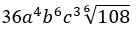# CBSE Worksheet for chapter-1 Number System class 9

## Find CBSE Worksheet for chapter-1 Number System class 9

CLASS-9

BOARD: CBSE

Mathematic Worksheet - 1

TOPIC: Number System

For other CBSE Worksheet for class 9 Mathematic check out main page of Physics Wallah.

#### SUMMARY

• REAL NUMBERS: Number which can represent its actual physical quantities in a meaningful way is called as real number.
• PRIME NUMBERS: All the numbers that have one and itself only as their factor is called as prime number.
• COMPOSITE NUMBER: All natural number which are not prime are composite numbers.
90-=O

#### EUCLID’S DIVISION LEMMA

Let a and b be any two positive integer. Then, there exists unique integers q and r such that a= bq + r, where +0≤r≤b.If

## Solutions: to worksheet-1 Topic-Number System

1. a
2. b
3. c
4. c
5. c

#### Subjective:

2) 0.110101001000100001
5)6)19/30